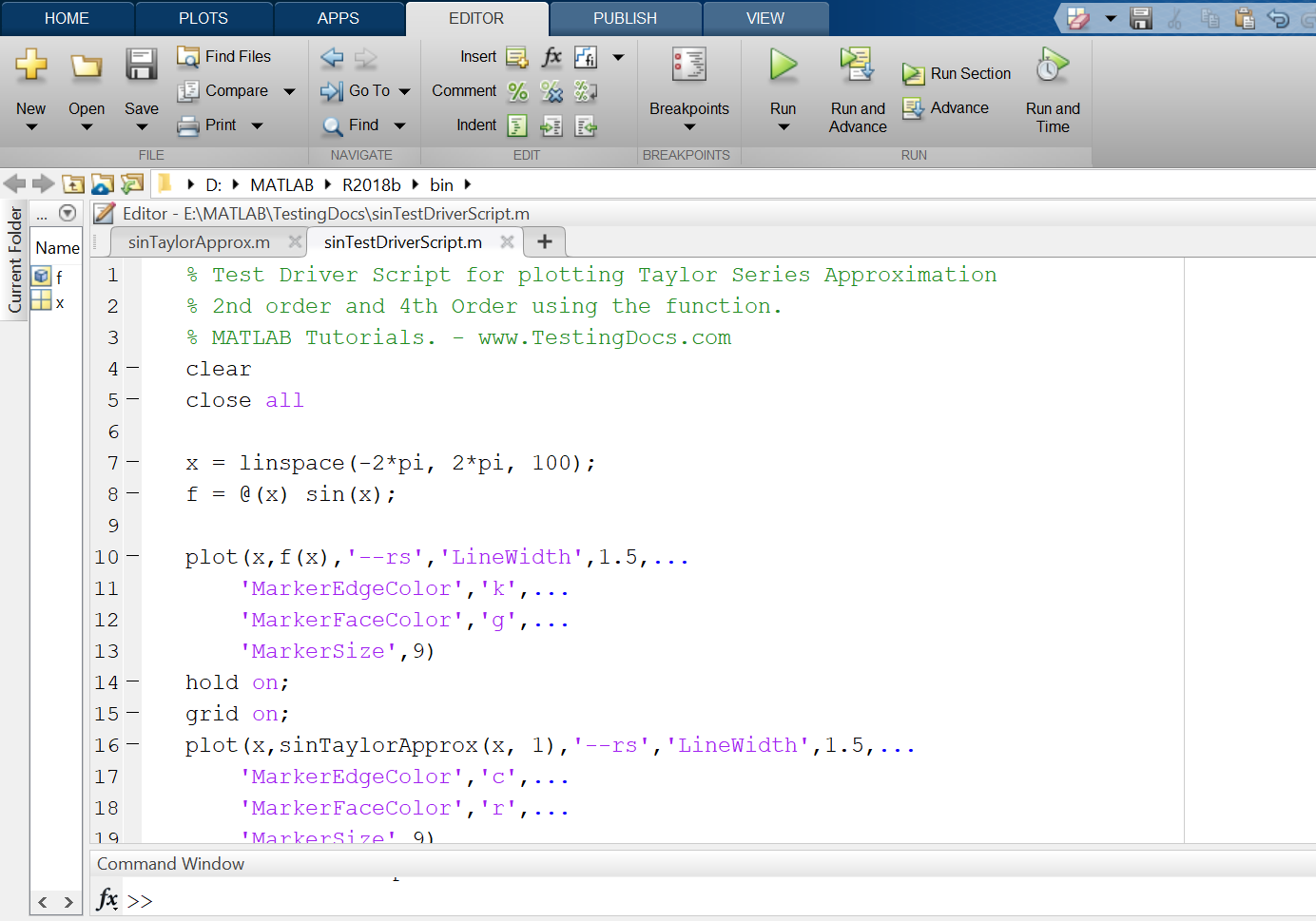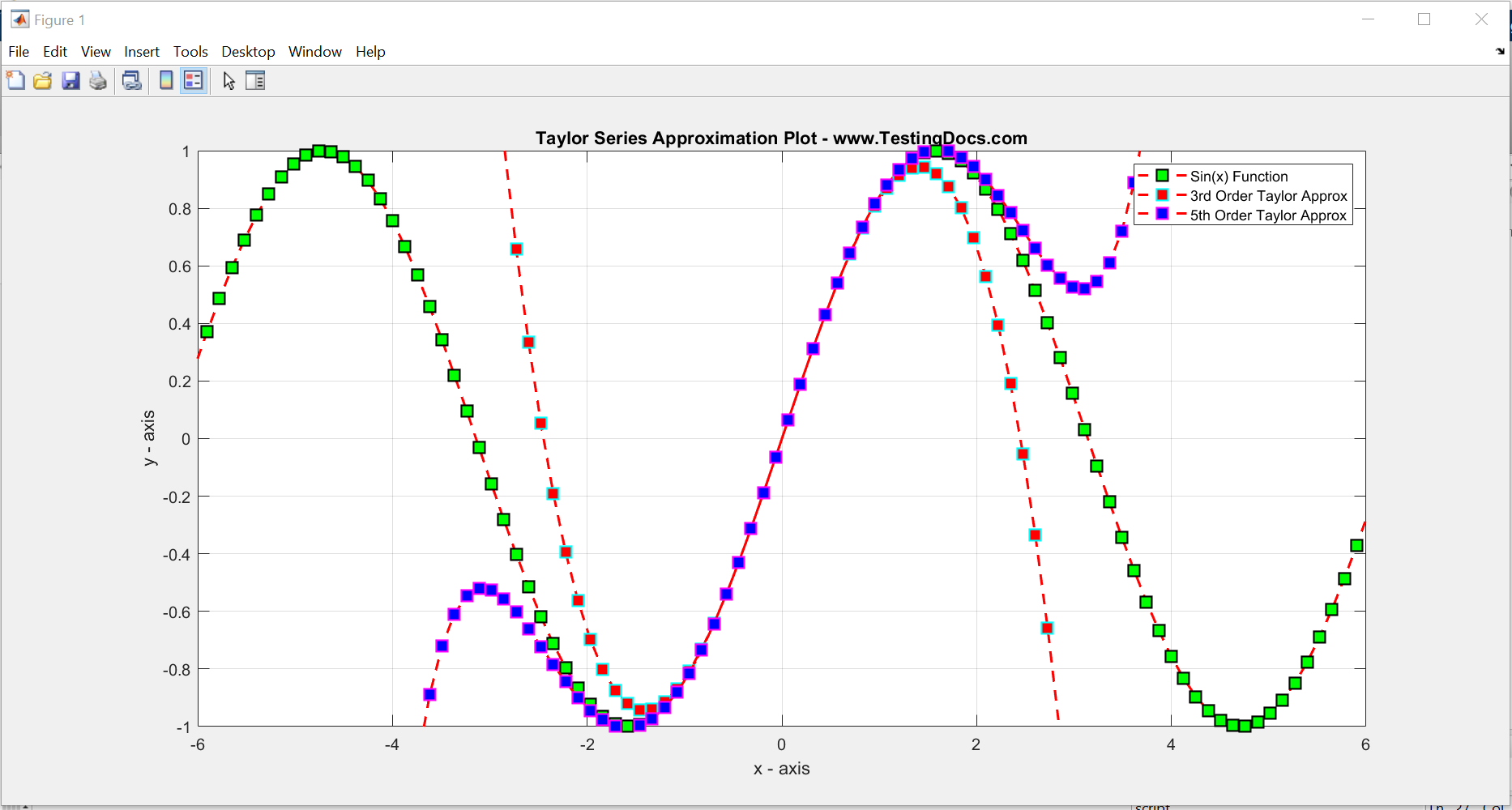# Introduction

In this post, we will learn MATLAB program to compute the Taylor series approximation of a simple function like sin(x). In fact, we can use this approximations to other functions and polynomials.

## Taylor Series

Taylor series of a function f(x) is an infinite series at a point a and can be written as :

where

is the nth derivative of the function at point a. The series is also called Maclaurin series when a = 0.

### Example

Let’s compute the Taylor series for sin(x) at point a = 0.

Apply the Taylor series expansion formula:

For better understanding of the series lets calculate each term individually for first few terms

The first tern would be =

Second term in the series =

The derivative of sin(x) = cos(x)

The third term in the series =

The second derivative of sin(x) = -sin(x)

The fourth term in the series =

We can ignore the even terms that contain the sin(0) in the series as sin(0) = 0.

We can generalize the series as :

Note that:  cos(0) = 1

## MATLAB Program

### Function

function [out] = sinTaylorApprox(x, N)
% Input = x, N
% out vector : Taylor series approx of sin(x)
out=zeros(1,numel(x));

for i = 1 : numel(x)
for k = 0: N
out(i) = out(i)+ ( (-1)^k * (x(i))^(2*k + 1) ) / factorial(2*k + 1);
end
end
end

## Test Driver Script

Using the defined function to compute the Taylor series approximation for the sin(x), we will create a driver script to plot the graphs for 3rd and 5th order approximations.

The driver script invokes the function twice with input arguments.


% Test Driver Script for plotting Taylor Series Approximation
% 2nd order and 4th Order using the function.
% MATLAB Tutorials. - www.TestingDocs.com
clear
close all

x = linspace(-2*pi, 2*pi, 100);
f = @(x) sin(x);

plot(x,f(x),'--rs','LineWidth',1.5,...
'MarkerEdgeColor','k',...
'MarkerFaceColor','g',...
'MarkerSize',9)
hold on;
grid on;
plot(x,sinTaylorApprox(x, 1),'--rs','LineWidth',1.5,...
'MarkerEdgeColor','c',...
'MarkerFaceColor','r',...
'MarkerSize',9)
plot(x,sinTaylorApprox(x, 2),'--rs','LineWidth',1.5,...
'MarkerEdgeColor','m',...
'MarkerFaceColor','b',...
'MarkerSize',9)
xlabel('x - axis');
ylabel('y - axis');
axis([-6 6 -1 1])
legend('Sin(x) Function', '3rd Order Taylor Approx','5th Order Taylor Approx');
title('Taylor Series Approximation Plot - www.TestingDocs.com');
-## Approximations GraphsMATLAB Tutorials on this website can be found here:

https://www.testingdocs.com/matlab-tutorial/